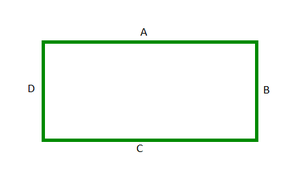# Java Program to Find the Perimeter of a Rectangle

• Last Updated : 22 Dec, 2021

A Rectangle is a quadrilateral with four right angles (90°). In a rectangle, the opposite sides are equal. A rectangle with all four sides equal is called a Square. A rectangle can also be called as right angled parallelogram.Rectangle

In the above rectangle, the sides A and C are equal and B and D are equal.

The perimeter of a rectangle is the total length of all its four sides. It can be simply calculated by all its four sides.

`Perimeter of rectangle ABCD = A+B+C+D`

Since the opposite sides are equal in a rectangle, it can be calculated as the sum of twice of one of its side and twice of its adjacent side.

`Perimeter of rectangle ABCD = 2A + 2B = 2(A+B)`

Program

## Java

 `// Java program to find the perimeter of a Rectangle`` ` `import` `java.io.*;`` ` `class` `GFG {``   ` `    ``// Method to calculate the perimeter of the rectangle``    ``// with given length and breadth``    ``static` `void` `perimeter(``int` `length, ``int` `breadth)``    ``{``        ``// Calculate the 'perimeter' using the formula``        ``int` `perimeter = ``2` `* (length + breadth);``       ` `        ``System.out.println(``"The perimeter of the given rectangle of length "``            ``+ length + ``" and breadth "` `+ breadth + ``" = "``            ``+ perimeter);``    ``}`` ` `    ``// Driver method``    ``public` `static` `void` `main(String[] args)``    ``{``        ``// Initialize a variable length that stores length of``        ``// the given rectangle``        ``int` `length = ``10``;``       ` `        ``// Initialize a variable breadth that stores breadth``        ``// of the given rectangle``        ``int` `breadth = ``20``;``       ` `        ``// Call the perimeter method on these length and``        ``// breadth``        ``perimeter(length, breadth);``    ``}``}`
Output
`The perimeter of the given rectangle of length 10 and breadth 20 = 60`
My Personal Notes arrow_drop_up